Performance
51.92k points

Description
The Core 2 Quad Q8400 is a cpu manufactured by Intel that was released on April 2009. This model has 4 Yorkfield cores , runs at 2666 MHz as base frequency and has a a thermal design power of 95 W.

Specs
Official name
Intel(R) Core(TM)2 Quad CPU Q8400 @ 2.66GHz
CPUID
1067a
Core
Yorkfield
Architecture
Base frecuency
2.666 GHz
Boost frecuency
2.666 GHz
Socket
LGA 775
4/4
TDP
95 W
Cache L1 (d+i)
128+128 kB
Cache L2
4096 kB
Cache L3
0 kB
Release date
April 2009
14.04k points
51.92k points

Non-optimized benchmark
The benchmark in Mode 0 (FPU) measures cpu performance with non-optimized software. It uses the basic µinstructions from the i386 architecture with the i387 floating point unit. This mode is compatible with all CPUs so it's practical to compare very different CPUs
Test#1 (Integers)
2.44k
9.71k (x4.0)
Test#2 (FP)
6.24k
24.81k (x4.0)
Test#3 (Generic, ZIP)
2.62k
10.51k (x4.0)
Test#1 (Memory)
1.43k
2.03k (x1.4)
TOTAL
12.73k
47.05k (x3.7)

SSE3 optimized benchmark
The benchmark in mode I (SSE) is optimized for the use of SIMD instructions with 128 bits register and the SSE set up to version 3. Nearly every modern CPU has support for this mode.
Test#1 (Integers)
3.08k
12.25k (x4.0)
Test#2 (FP)
6.8k
26.84k (x3.9)
Test#3 (Generic, ZIP)
2.73k
10.82k (x4.0)
Test#1 (Memory)
1.42k
2.02k (x1.4)
TOTAL
14.04k
51.92k (x3.7)

Computers where this CPU was tested
Model
Release date

RAM models tested along with this CPU
Model
Type
Frequency
Size
DDR3
1333 MHz
2 GB

Monothread performance graphics gives the performance vs time. They are useful to measure the time it takes to the CPU to reach the maximum performance.

Usually, CPU's performance will be steady during these tests but if it has a slow frequency strategy, the first samples will show a lower score.

Test#1 (Integers) [% vs time]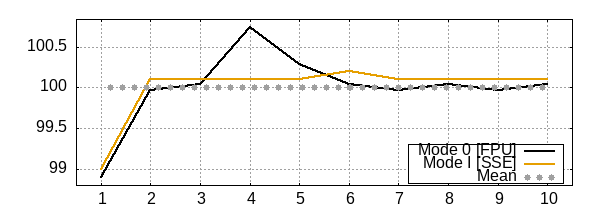Test#2 (FP) [% vs time]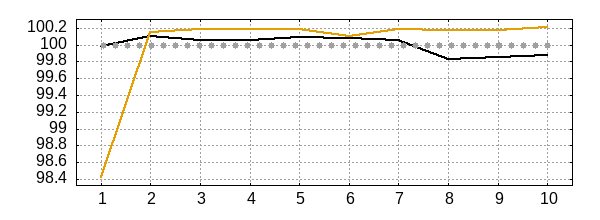Test#3 (Generic, ZIP) [% vs time]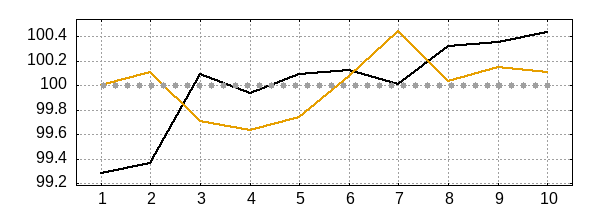Test#1 (Memory) [% vs time]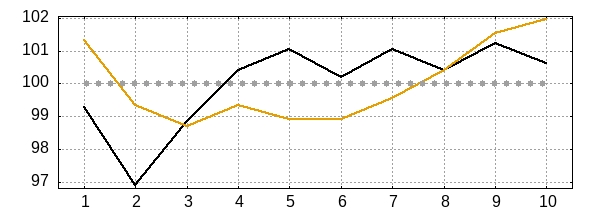Multithread graphs measure the performance against a heavy load during certain time.

If CPU's TDP doesn't limit the frequency and the machine is properly cooled, performance should remain steady vs time. Otherwise, the performance score will oscillate or decrease over time.

Test#1 (Integers) [% vs time]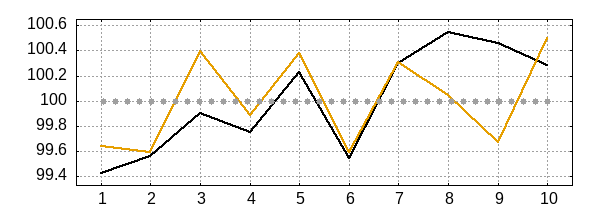Test#2 (FP) [% vs time]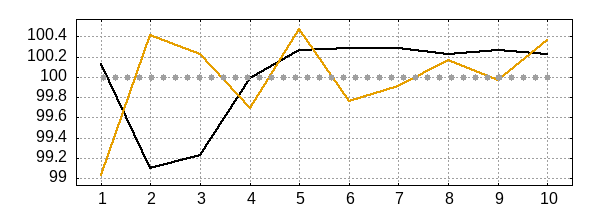Test#3 (Generic, ZIP) [% vs time]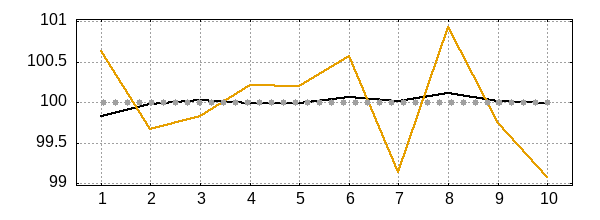Test#1 (Memory) [% vs time]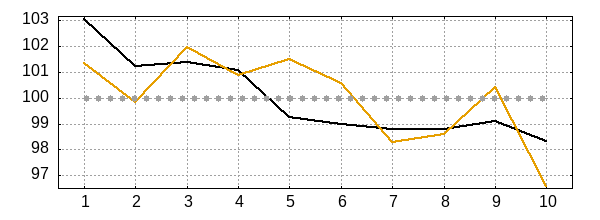Similar CPUs to Core 2 Quad Q8400
Core 2 Quad Q8400 (This model)
Mode FPU monothread  [12.75k points +0.15%]
Mode FPU multithread  [24.2k points -48.58%]
Mode SSE monothread  [14.01k points -0.21%]
Mode SSE multithread  [26.78k points -48.42%]
Mode FPU monothread  [16.42k points +29.03%]
Mode FPU multithread  [48.96k points +4.05%]
Mode SSE monothread  [17.29k points +23.18%]
Mode SSE multithread  [48.98k points -5.66%]
Mode SSE monothread  [15.62k points +11.32%]
Mode SSE multithread  [51.64k points -0.54%]
Mode FPU monothread  [12.23k points -3.87%]
Mode FPU multithread  [22.76k points -51.63%]
Mode SSE monothread  [13.46k points -4.08%]
Mode SSE multithread  [25.21k points -51.44%]
Mode FPU monothread  [13.66k points +7.34%]
Mode FPU multithread  [25.29k points -46.26%]
Mode SSE monothread  [14.32k points +2.06%]
Mode SSE multithread  [25.87k points -50.17%]
Mode SSE monothread  [15.77k points +12.34%]
Mode SSE multithread  [25.39k points -51.10%]
Mode FPU monothread  [15.39k points +20.91%]
Mode FPU multithread  [44.38k points -5.69%]
Mode SSE monothread  [16.62k points +18.40%]
Mode SSE multithread  [48.62k points -6.36%]
Mode FPU monothread  [13.16k points +3.44%]
Mode FPU multithread  [41.57k points -11.65%]
Mode SSE monothread  [18.22k points +29.84%]
Mode SSE multithread  [61.49k points +18.44%]
Mode FPU monothread  [16.55k points +30.05%]
Mode FPU multithread  [23.82k points -49.38%]
Mode SSE monothread  [17.22k points +22.66%]
Mode SSE multithread  [27.21k points -47.60%]
Mode FPU monothread  [11.89k points -6.62%]
Mode FPU multithread  [22.03k points -53.18%]
Mode SSE monothread  [13.08k points -6.80%]
Mode SSE multithread  [24.89k points -52.07%]

FPU mode comparison

Model
Score
2.44k
This model
2.41k [-1.41%]

Model
Score
11.66k [20.04%]
11.6k [19.48%]
10.78k [10.97%]
9.71k
This model
9.67k [-0.47%]
9.19k [-5.36%]
9.06k [-6.68%]
9.05k [-6.79%]

Model
Score
6.24k
This model
5.85k [-6.25%]

Model
Score
25.55k [2.97%]
25.28k [1.92%]
24.81k
This model
24.15k [-2.64%]
23.09k [-6.93%]
23.04k [-7.12%]
22.92k [-7.63%]
22.82k [-8.00%]

Model
Score
2.62k
This model
2.56k [-2.11%]
2.52k [-3.65%]

Model
Score
11.48k [9.27%]
11.28k [7.32%]
10.82k [2.95%]
10.59k [0.76%]
10.51k
This model
10.26k [-2.33%]
10.25k [-2.43%]
10.22k [-2.71%]
9.7k [-7.70%]

Model
Score
1.65k [15.57%]
1.61k [12.56%]
1.43k
This model

Model
Score
2.45k [20.86%]
2.44k [20.25%]
2.43k [20.05%]
2.27k [11.93%]
2.12k [4.35%]
2.03k
This model
2.01k [-0.84%]
1.74k [-14.21%]

SSE mode comparison

Model
Score
3.09k [0.32%]
3.08k
This model

Model
Score
12.56k [2.58%]
12.54k [2.39%]
12.4k [1.21%]
12.27k [0.20%]
12.25k
This model
11.78k [-3.85%]
11.25k [-8.16%]
11.19k [-8.65%]

Model
Score
6.86k [0.91%]
6.8k
This model

Model
Score
28.17k [4.95%]
27.61k [2.88%]
27.45k [2.26%]
27.27k [1.59%]
26.84k
This model
25.71k [-4.22%]
25.44k [-5.21%]
24.85k [-7.41%]

Model
Score
2.73k
This model
2.73k [-0.10%]
2.69k [-1.44%]

Model
Score
12.01k [11.04%]
11.96k [10.60%]
11.74k [8.54%]
11.24k [3.93%]
10.82k
This model
10.81k [-0.08%]
10.58k [-2.21%]
10.41k [-3.78%]
10.35k [-4.29%]
10.15k [-6.18%]

Model
Score
1.45k [2.58%]
1.43k [0.58%]
1.42k
This model
1.39k [-1.78%]
1.37k [-3.52%]
1.32k [-6.60%]